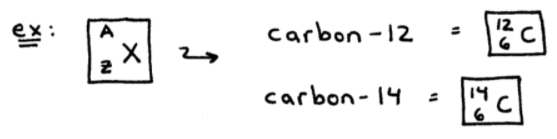# S19E1 - The Nucleus, Nuclear Stability and Radioactive Decay

The nucleus is not of primary importance to chemists because the chemistry of atoms is generally determined by the number and arrangements of their electrons.

And electrons of course, are found outside of the nucleus.

But the nucleus and its chemistry has a big impact on our society.

==========

## The Nucleus

➞  contains protons and neutrons.

➞  is very small

What is meant by "small" you say?...

----------

## Size of the Nucleus

Well a nuclear radius is roughly 10-13 cm.

If a nucleus were the size of a ping-pong ball, the nearest electron (the 1s1 e-) would be 0.30 miles away!

In addition to being extremely small, the nucleus is also very dense.

----------

## Density of the Nucleus

➞  density  =  on average: 1.6 x 1014 g/cm3 !!

Compare this to something we think as being "really dense"  =  lead (Pb), which is 11.3 g/cm3.

Density of Lead Weight:  11.3 g/cm3
vs.
Density of Nucleus:  160,000,000,000,000 g/cm3That "ping-pong ball" sized nucleus we mentioned earlier?

Um.... it would weigh 2.5 million tons!!

==========

## Nuclear Chemistry  -  Key Terms

The energies involved in nuclear processes (reactions) are millions of times larger than typical chemical reactions

Nucleons  =  a particle in the atomic nucleus; either a proton or a neutron.

Atomic Number (Z)  =  the total number of protons.

Mass Number (A)  =  sum of the number or protons and the number of neutrons.

Isotopes  =  atoms of the same element, having the same number of protons but different numbers of neutrons.Nuclide  =  term used to describe a particular isotope.

In the example above, carbon-14 is a nuclide of carbon.

==========

## Nuclear Stability

Many nucleii are radioactive, which means they can decompose, forming another nucleus and producing one or more other particles.

Here's an example of a typical radioactive decay reaction...

----------Notice that the Z and A values must be conserved on each side of the reaction arrow.

➞  On the left and on the right, A = 14 (total).

➞  On the left and on the right, Z = 6 (total).

==========

In my next post covering SECTION 19 - The Nucleus and Nuclear Chemistry,